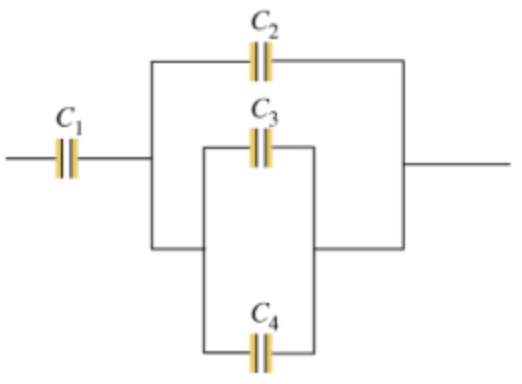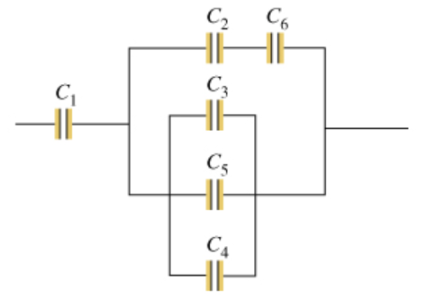# Problem: A) Consider the combination of capacitors shown in the diagram, where C1 = 3.00 μF, C2 = 11.0 μF, C3 = 3.00 μF, and C4 = 5.00 μF.Find the equivalent capacitance CA of the network of capacitors. Express your answer in microfarads.B) Two capacitors of capacitance C5 = 6.00 μF and C6 = 3.00 μF are added to the network, as shown in the diagram. Find the equivalent capacitance CB of the new network of capacitors.

###### FREE Expert Solution

For capacitors in parallel:

$\overline{){{\mathbf{C}}}_{\mathbf{e}\mathbf{q}}{\mathbf{=}}{{\mathbf{C}}}_{{\mathbf{1}}}{\mathbf{+}}{{\mathbf{C}}}_{{\mathbf{2}}}{\mathbf{+}}{\mathbf{.}}{\mathbf{.}}{\mathbf{.}}{\mathbf{+}}{{\mathbf{C}}}_{{\mathbf{n}}}}$

For series:

$\overline{){{\mathbf{C}}}_{\mathbf{e}\mathbf{q}}{\mathbf{=}}\frac{{\mathbf{C}}_{\mathbf{1}}{\mathbf{C}}_{\mathbf{2}}}{{\mathbf{C}}_{\mathbf{1}}\mathbf{+}{\mathbf{C}}_{\mathbf{2}}}}$

(A)

C3 and C4 are in parallel.

C34 = 3.00 + 5.00 = 8.00 μF

C2 and C34 are in parallel.

C234 = 11.0 + 8.00 = 19.0 μF###### Problem Details

A) Consider the combination of capacitors shown in the diagram, where C1 = 3.00 μF, C2 = 11.0 μF, C3 = 3.00 μF, and C4 = 5.00 μF.B) Two capacitors of capacitance C5 = 6.00 μF and C6 = 3.00 μF are added to the network, as shown in the diagram. Find the equivalent capacitance CB of the new network of capacitors.